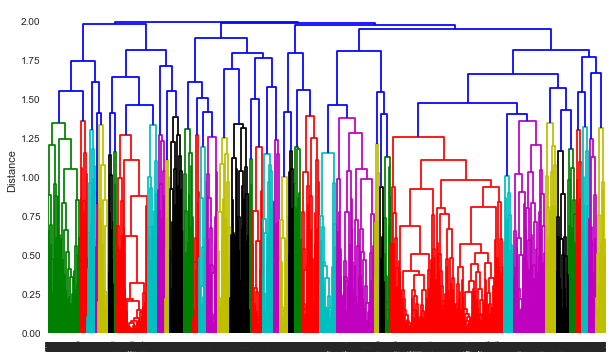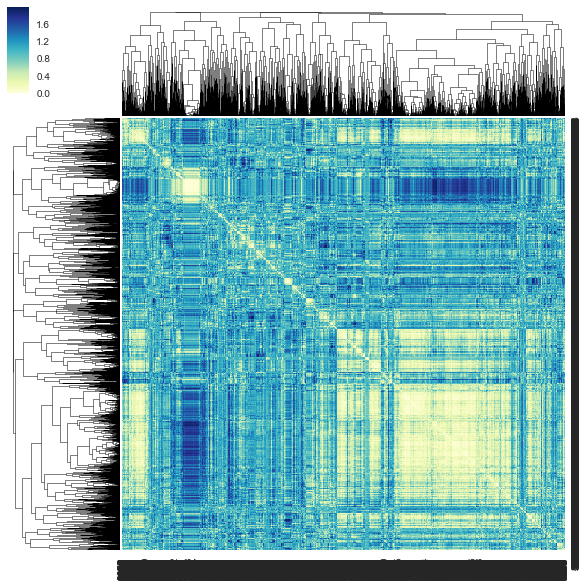# Clustering of metabolite peaks from LC-MS¶

Incorporating retention time into hierachical clustering of LC-MS peaks.

This was first used in Gardinassi et al. (2018) Integrative metabolomics and transcriptomics signatures of clinical tolerance to Plasmodium vivax reveal activation of innate cell immunity and T cell signaling. Redox Biology. DOI: 10.1016/j.redox.2018.04.011

The example below is part of data from the paper.

Shuzhao Li, 2018-05-12

In :
import os
import pandas as pd
import numpy as np

import matplotlib.pyplot as plt
import seaborn as sns

%matplotlib inline

from scipy.cluster.hierarchy import *
from scipy.spatial.distance import pdist, squareform

infile = "../input_data/HILIC_pos_diag_basel.txt"

In :
metabo = pd.read_table(infile)
print(metabo.shape)

(4236, 13)

Out:
mz_rt N1 N2 N3 N4 N5 N6 SI1 SI2 SI3 SI4 SI5 SI6
0 85.02865196_275.3154044 -0.741169 -1.394805 -0.620273 0.857773 -1.613470 -0.264445 -1.909026 -0.187610 1.388254 0.878922 -0.934093 2.638685
1 85.02868748_114.3203084 -2.711312 1.161496 5.581955 -0.188379 -0.081080 0.621589 -0.875584 -0.542016 0.730462 1.173231 0.587078 7.605417
2 85.04799534_127.0756394 -0.059953 -5.980863 -0.026952 0.000000 -21.934977 -0.461426 -1.451391 -0.965788 0.979416 0.767319 -0.773038 -21.085978
3 85.06507359_261.7958538 -0.589275 -0.497958 0.441288 -1.408106 0.203421 -1.705279 -0.614097 -1.570598 0.656213 0.304973 0.163556 0.462155
4 85.07627944_160.8882793 -0.348159 -0.509134 -0.330221 0.701595 0.679800 -2.164348 -0.705403 -1.027574 1.476842 0.516237 -0.505817 1.322065
In :
'''
Default input format: m/z  retention_time  samples
# Scipy implementation of hierarchical clustering is mirroring Matlab
# https://docs.scipy.org/doc/scipy/reference/cluster.hierarchy.html
# https://www.mathworks.com/help/stats/hierarchical-clustering.html
This adds a function to penalize distance in retention time.
Output includes two figures, dendrogram and heatmap, which can be slow especially in pdf.
Sensitive to missing input data.
'''

# distance matrix, this is [1 - (Pearson R)]
YM = pdist(metabo.values[:, 1:], 'correlation')

retention_time = metabo.values[:,1]
min_retention_time, max_retention_time = min(retention_time), max(retention_time)
range_retention_time = max_retention_time - min_retention_time
print("min_retention_time, max_retention_time", min_retention_time, max_retention_time)

PearsonR = 1 - YM
delta_RT = []
for ii in range(metabo.shape):
for jj in range(ii+1, metabo.shape):
delta_RT.append(abs(retention_time[ii] - retention_time[jj]))

print("Vector delta_RT len: ", len(delta_RT))

#
# weighting function
# distance = 1 - (1 - delta_RT/range_retention_time)*PearsonR
#
YM_new = 1 - (1- np.array(delta_RT)/range_retention_time)*PearsonR

print("Shape of dist matrix, ", YM_new.shape)

# Metabolite features linkage matrix using new distance matrix

plt.figure(figsize=(10, 6))
#plt.title('HCL HILICpos study xyz')
plt.ylabel('Distance')
dendrogram(ZM)

# use .pdf if desired, but slower
plt.savefig('fig1.png')

('min_retention_time, max_retention_time', -26.600752399999998, 27.56155862)
('Vector delta_RT len: ', 8969730)
('Shape of dist matrix, ', (8969730,))In :
# Based on the dendrogram above, choose
distance_cut=1.5

plt.figure(figsize=(10, 10))
plt.savefig('fig2.png')

metClus = fcluster(ZM, distance_cut, criterion='distance')
# Compile clusters
metClusDict = {}
for ii in range(len(metClus)):
if metClusDict.has_key(metClus[ii]):
metClusDict[ metClus[ii] ].append(ii)
else:
metClusDict[ metClus[ii] ] = [ii]

print("number of clusters: ", len(metClusDict.keys()))

/Users/sli/anaconda2/lib/python2.7/site-packages/matplotlib/cbook.py:136: MatplotlibDeprecationWarning: The axisbg attribute was deprecated in version 2.0. Use facecolor instead.
warnings.warn(message, mplDeprecation, stacklevel=1)

('number of clusters: ', 31)

<matplotlib.figure.Figure at 0x10fd5f750>In :
# write out clusters.
def write_cluster(OUTDIR, wanted_clusters, metClus, metabo, prefix="metabo_"):
for c in wanted_clusters:
goodrows = []
for ii in range(metabo.shape):
if metClus[ii] == c:
goodrows.append(ii)

metabo.iloc[goodrows, :].to_csv( OUTDIR + prefix + "clus_%d.txt" %c, sep="\t")

# do all
wanted = metClusDict.keys()
# Need create OUTDIR first
OUTDIR = 'export_clusters/'
os.mkdir(OUTDIR)

write_cluster(OUTDIR, wanted, metClus, metabo, prefix="metabo_")

In :
# to export collapsed values of each cluster, as per sample a = sum(z score)/squareroot(feature number)
def zscore(V):
# input np array
V = list(V)
m, std = np.mean(V), np.std(V)
return (V-m)/std

def get_cluster_activity(M):
sqN = np.sqrt(M.shape)
new, new2 = [], []
for row in M:
new.append(zscore(row))
for ii in range(len(row)):
new2.append(sum([x[ii] for x in new])/sqN)

return new2

def write_cluster_activities(OUTDIR, wanted_clusters, metClus, metabo, prefix="metabo_"):
'''
To export collapsed values of each cluster, as per sample a = sum(z score)/squareroot(feature number)
The columns of m/z and rtime may bet converted but they are meaningless
'''
s = 'cluster_number\t' + '\t'.join(list(metabo.columns)) + '\n'
for c in wanted_clusters:
goodrows = []
for ii in range(metabo.shape):
if metClus[ii] == c:
goodrows.append(ii)
# Note values in metabo starts from col 1
s += prefix+str(c) + '\t' + '\t'.join(
[str(x) for x in get_cluster_activity(metabo.iloc[goodrows, 1:].values)]) + '\n'

with open('cluster_activities.txt', 'w') as file:
file.write(s)

write_cluster_activities('./', wanted, metClus, metabo, prefix="metabo_")


The output cluster activities can be used for further statistical analysis, similarly to feature level analysis.